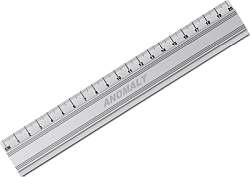# Centimeters to Feet Calculator

Instructions: Use this calculator to convert a centimeters to feet, showing all the steps. Please type the number of centimeters you would like to convert in the form below:Type the number of centimeters:

## Using this Centimeter to Feet Conversion Calculator

Metric conversions are quite useful. If a German person is talking to someone from the US, and asked "how tall are you?" she would respond something like "172 cms" or "1.72 meters", and the person in US maybe a bit clueless and would ask back "How much is that in feet and inches?"

So, within our own local context, perhaps very unusually will we need conversions, but as soon as we cross borders, necessity may arise, and you would definitely like for example to convert heights in feet and inches to centimeters, or perhaps to meters.## How many centimeters are there in a foot?

Basic unit equivalence shows that 1 foot is approximately the same as 30.48 centimeters. This equivalence between fundamental units will be crucial at the time of making a general conversion.

## How do you convert centimeters to feet?

Converting units is a simple process, and it involves the application of direct proportionality, which leads to a simple conversion formula.

### Steps for the conversion of centimeters to Feet

1. Notice that 1 foot is the same as 30.48 centimeters. We will use this as the basic starting proportion
2. Assume you have given C centimeters. You want to know many feet F are equivalent to those C centimeters
3. The direct proportion that needs to be used is $$\displaystyle \frac{1}{30.48} = \frac{F}{C}$$
4. Solving the proportion for F, we get the following conversion formula: $$F = \displaystyle \frac{C}{30.48}$$

Then, after you have the formula for converting cms to feet, all you need to do is to plug in the corresponding number of centimeters you want to convert.### Examples: Converting centimeters to feet

What is 173 cms in feet?

Solution: Using the conversion formula:

$F = \displaystyle \frac{C}{30.48}$

We need to plug the value of C = 173 cms in the above formula, so we get:

$F = \displaystyle \frac{C}{30.48} = \displaystyle \frac{173}{30.48} = 5.676 \text{ feet}$

### Another conversion example

Convert 190 cms in feet

Solution: Exactly the same procedure. Using the conversion formula:

$F = \displaystyle \frac{C}{30.48}$

we need get C = 190 cms and plug it in the above formula, so we get:

$F = \displaystyle \frac{C}{30.48} = \displaystyle \frac{190}{30.48} = 6.234 \text{ feet}$

### Example

Convert 170 cms to feet

Solution: You get the idea: Identify the number of centimeters you want to convert. You will use the following conversion formula:

$F = \displaystyle \frac{C}{30.48}$

and in this case the number of centimeters we want to convert are C = 170 cms. We plug this in:

$F = \displaystyle \frac{C}{30.48} = \displaystyle \frac{170}{30.48} = 5.577 \text{ feet}$

### Another conversion example

Convert 2 centimeters to feet

Solution: In this case the number of centimeters we want to convert are C = 2 cms. We plug this in:

$F = \displaystyle \frac{C}{30.48} = \displaystyle \frac{2}{30.48} = 0.0656 \text{ feet}$

## Conversion calculators

Other conversion calculators you may be interested in are our meters to inches calculator, or the centimeters to inches, meters to feet, imperial to centimeters or imperial to meters calculators, according to the type of conversion that suits your needs.

One of the great advantages of our calculators is that they show all the steps of the process.